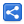# Python Keywords

[Last Updated: Feb 2, 2022]

In a programming language keywords are reserved words. These words are used as the programming constructs and they have special meanings to the compiler. Keywords are part of the syntax and they cannot be used as identifiers (variable/function/class etc names)

Following is the list of Python keywords with example snippets:

Keyword Description Example
True / False
Boolean literals
```a = True
b = False```
and / or / not
Logical operators
```a = True
b = False
print(a and b)
print(a or b)
print(not b)

```
```False
True
True```
as
To create an alias
For example importing a module:
`import math as calc`
assert
It's used a condition to make sure a condition is always true. It is mainly used in tests or debugging purpose.
Following will run fine:
```s = 'hello!'
assert (len(s) > 1)
```
Following will end in an error:
```s = ''
assert (len(s) > 1)```
```........
assert (len(s) > 1)
AssertionError
```
for / break / continue
Creating /terminating / continuing loops
```for x in range(3, 6):
if x % 4 == 0:
continue
if x % 2 == 0:
break
print(x)```
`5`
class
To crate a class
```class Person:
name = 'joe'
p = Person()
print(p.name)```
`joe`
def
To define a function
```def multiply(x, y):
print(x * y)
multiply(3, 5)```
`15`
del
To delete an object
```class Person:
name = 'joe'
p = Person()
del p
print(p)```
```NameError:
name 'p' is not defined```
if /elif / else
To create conditional statement
```x = 3
if x < 3:
print("less than 3")
elif x > 3:
print("more than 3")
else:
print("exactly 3")
```
`exactly 3`
import / from
Importing module / Importing specific part of module
`from math import pi`
in
(a) To iterate a list
(b) To check if an element is contained by a list
(a) To iterate:
```num = [10, 20]
for x in num:
print(x)```
```10
20```
(b) To check element is in the list:
```num = [10, 20]
result = 10 in num
print(result)```
`True`
is
To test if two variable are equal
```x = 2
y = 3
result = x is y
print(result)```
`False`
lambda
To create anonymous functions
```a = lambda x: print(x)
a(5)```
`5`
None
To define a null variable
```a = None
print(a)```
`None`
nonlocal
To declare a non-local variable which allows to change variables defined outside of the current scope
```def num():
x = 3
def num2():
nonlocal x
x = 5
num2()
return x
print(num())
```
`5`
If we remove line `nonlocal x` then the output will be 3.
global
To declare a global variable
```def run():
global x
x = 5
run()
print(x)```
`5`
pass
To use as empty code where empty blocks are not allowed
```def run():
pass```
raise
To raise an error
```name = ''
if len(name) == 0:
raise Exception("name cannot be empty")```
`Exception: name cannot be empty`
return
To return or exit a function
```def square(x):
if x < 0:
return
return x * x
print(square(3))
print(square(-3))```
```9
None```
while
To create a while loop
```x = 3
while x > 0:
print(x)
x = x - 1```
```3
2
1```
try / except / finally
To catch an error. finally block will always execute, even there's no error
```x = 0
try:
x = 5 / x
except ZeroDivisionError:
print("error")
finally:
print("end")```
```error
end```
with
Simplifies the use of try... finally
```with open('my-file', 'w') as file:
file.write('test') ```
yield
To create a generator that can be iterated
```def even():
for x in range(3, 8):
if x % 2 == 0:
yield x
for i in even():
print(i)
```
```4
6```

 Share